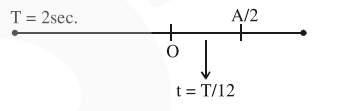# A particle performs simple harmonic motion`
Question:

A particle performs simple harmonic motion with a period of 2 second. The time taken by the particle to cover a displacement equal to half of its amplitude from the mean position

is $\frac{1}{\mathrm{a}} \mathrm{s}$. The value of 'a' to the nearest integer is___________.

Solution:$t=\frac{2}{12}=\frac{1}{6}$

$\therefore$ Correct answer $=6.00$